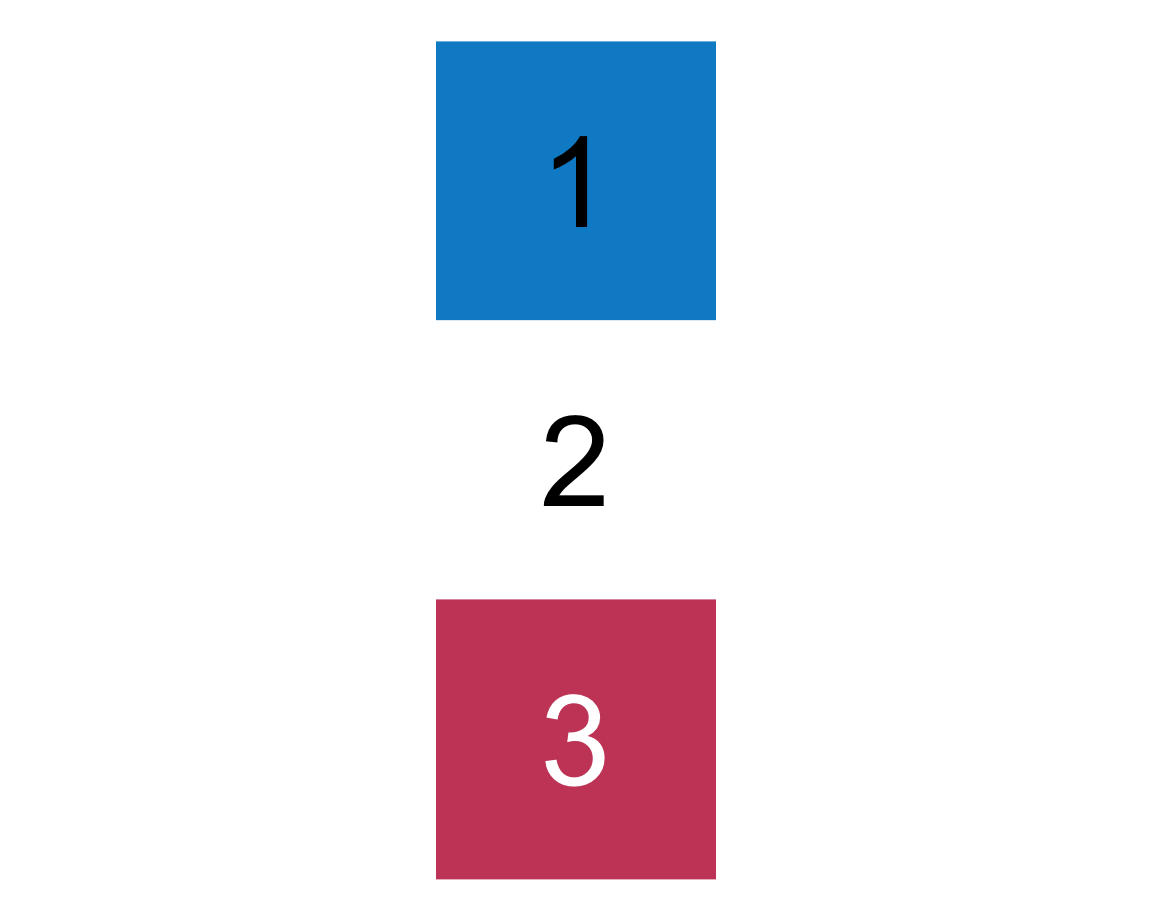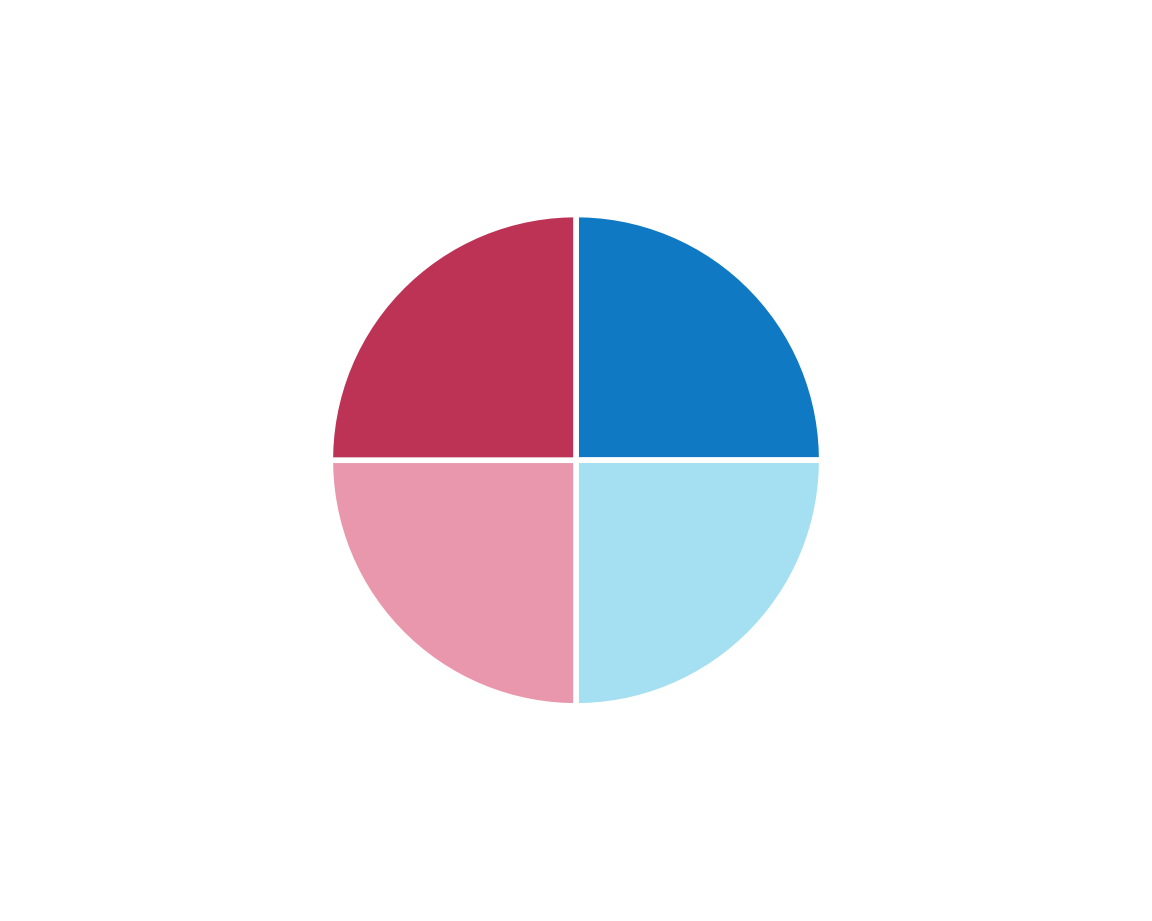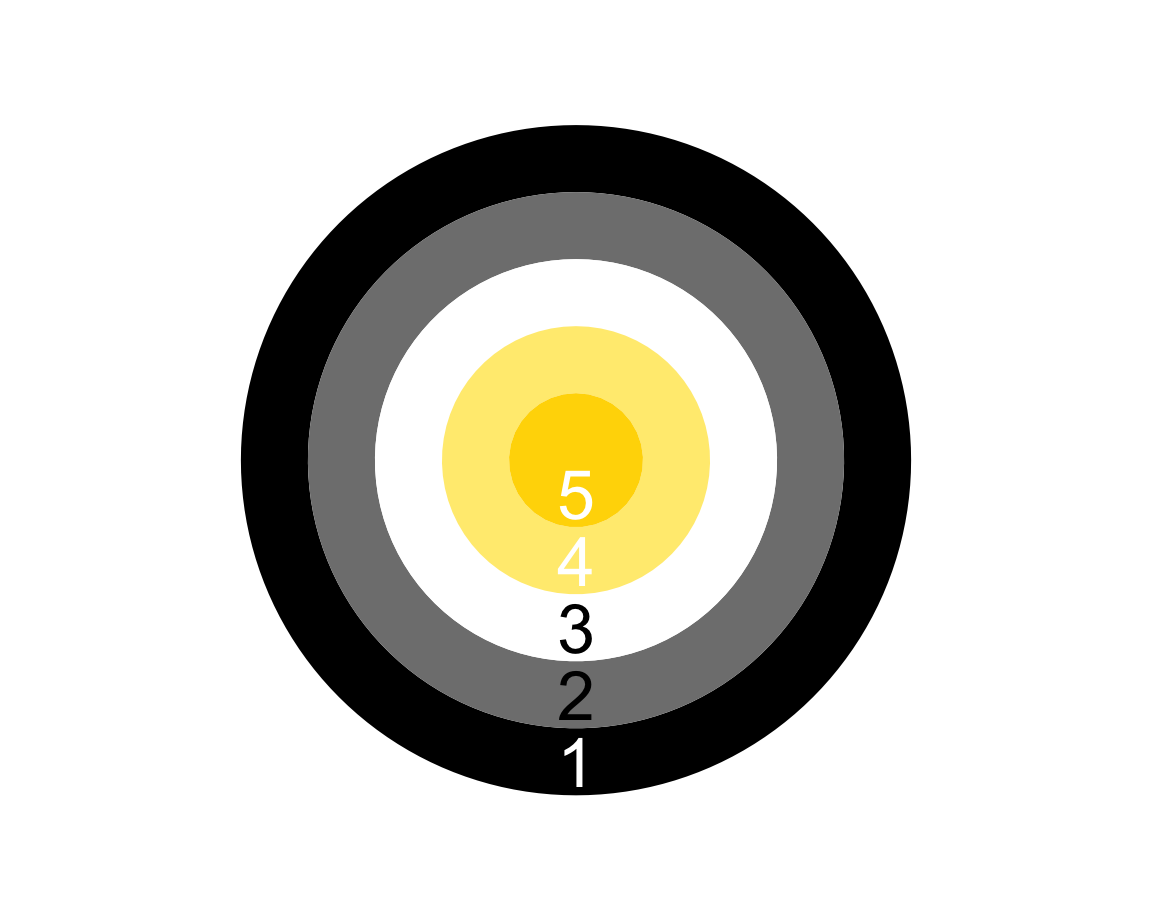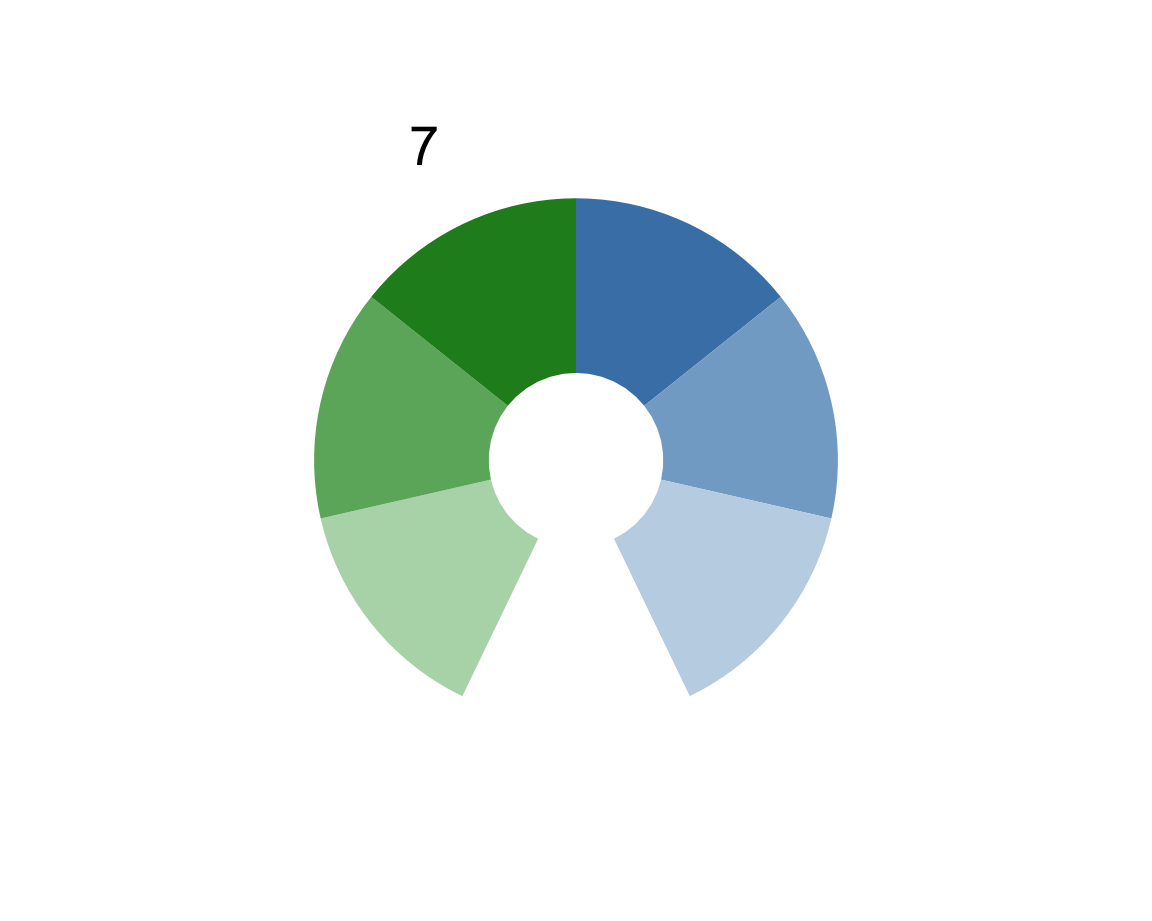## 2.4 Functions

Functions are R objects that serve as tools — they do stuff with data (as input) to often create new data (as output). Alternative terms for function — depending on context — are command, method, procedure, or strategy.

Theoretically, a function provides a mapping from one set of objects (e.g., inputs or $$x$$-values) to another set of objects (e.g., outputs or $$y$$-values). Internally, a function is a computer program that takes some input(s) and yields some output(s) or side-effects. Most functions are only a few lines of code long, but others may contain thousands of lines of code or call many other functions.

The great thing about functions is that they are tools that encapsulate processes — they are “abstraction devices.” Just like we usually do not care how some technological device (e.g., a phone) gets its task done, we can treat a function as a black box that is designed to perform some task. If we provide it with the right kind of input, a function typically does something and returns some output. If all works well (i.e., we get our desired task done), we never need to care about how a function happens to look or work inside.

R comes pre-loaded with perhaps a few hundred functions. However, one of the main perks of R is that more than 19,000 R packages contributed by R developers provide countless additional functions that someone else considered useful enough for creating and sharing it. So rather than ever learning all R functions, it is important that we learn how to find those that help us solving our problems and how to explore and use them in a productive fashion.9 As we get more experienced, we will also learn how to create our own R functions (by — surprise — using an R function). But before we do all that, we first need to learn how to use a function that already is provided to us.

We already encountered a large number and variety of functions for inspecting and using vectors:

• object inspection functions (like typeof(), mode(), length(), names(), or attributes())
• arithmetic and logical functions (like sum(), mean(), or isTRUE())
• vector creation functions (like c(), rep(), and seq())
• more sophisticated functions (like sample())

When first learning R, learning the language essentially consists in knowing and using new functions. Even infix operators <-, +, !, and : are actually implemented as functions.

#### Testing for missing values

An important function in R is is.na(): It tests whether an object is missing or contains any missing values:

is.na(x)
#>  FALSE FALSE FALSE
is.na(ms)
#>  TRUE

and returns logical value(s) of either TRUE or FALSE.

Note that even a missing R object (i.e., an object for which is.na() returns TRUE) needs to exist to be evaluated. Thus, the following would yield an error, unless a unicorn object existed in our current environment:

is.na(unicorn)

#### Getting help

Whenever we are interested in an existing function, we can obtain help on it by typing its name preceded by a question mark. For instance, if we wanted to learn how the substr() function worked, we would evaluate the following command in our Console:

?substr()  # yields documentation
?substr    # (works as well)

Do not be discouraged if some of the function’s documentation seems cryptic at first. This is perfectly normal — and as our knowledge of R grows, we will keep discovering new nuggets of wisdom in R’s very extensive help pages.10 And even when not understanding anything of a function’s documentation, trying out its Examples usually provides some idea what the function is all about.

### 2.4.1 Function arguments

Importantly, functions have a name and accept arguments (in round parentheses). For instance, a function fun_name() could have the following structure:

fun_name(x, arg_1 = 1, arg_2 = 99)

Arguments are named slots that allow providing inputs to functions. The value of an argument is either required (i.e., must be provided by the user) or is set to default value (which is used if the argument is not provided). In our example structure, the arguments x is required, but arg_1 and arg_2 have default values.

We use the substr() function to illustrate the use of arguments with an actual function. Evaluating ?substr() describes its purpose, arguments, and many details and examples for its usage. To identify an argument, we can use their name or the order of arguments:

substr(x = "perspective", start = 4, stop = 8)  # explicating argument names
#>  "spect"
substr("perspective", 4, 8)                     # using the order of arguments
#>  "spect"

Note that there is no space between the function name and the parentheses and multiple arguments are separated by commas. Although it is faster and more convenient to omit argument names, explicating argument names is always safer. For instance, calling functions with explicit argument names would still work if the author of a function added or changed the order of arguments:

substr(start = 4, x = "perspective", stop = 8)  # explicit names (in different order)
#>  "spect"

Note that a function’s documentation typically mentions the data types of its input(s), its output(s), and its argument(s). This is important, as most functions are designed to work with specific data types.

### 2.4.2 Exploring functions

As R and R packages contain countless functions, an important skill consists in exploring new functions. Exploring a new function is a bit like conducting a small research study. To be successful, we need a mix of theoretical guidance and empirical observations to become familiar with a new object. When exploring an R function, we should always ask the following questions:

• Purpose: What does this function do?
• Arguments: What inputs does it take?
• Outputs: Which outputs does it yield?
• Limits: Which boundary conditions apply to its use?

Note that we currently explore functions from a user’s perspective. When we later learn to write our own functions, we will ask the same questions from a designer’s perspective.

Example: The ds4psy package provides a function plot_fn() that is deliberately kept cryptic and obscure to illustrate how a function and its arguments can be explored. Most actual R functions will be easier to explore, but they also require some active exploration to become familiar with them. Here is what we normally do to explore an existing function:

library(ds4psy)  # load package
?plot_fn         # get documentation

The documentation (shown in the Help window of RStudio) answers most of our questions. It also provides some examples, which we can copy and evaluate for ourselves:

# Basics:
plot_fn()# Exploring options:
plot_fn(x = 2, A = TRUE)plot_fn(x = 3, A = FALSE, E = TRUE)plot_fn(x = 4, A = TRUE,  B = TRUE, D = TRUE)plot_fn(x = 5, A = FALSE, B = TRUE, E = TRUE, f = c("black", "white", "gold"))plot_fn(x = 7, A = TRUE,  B = TRUE, F = TRUE, f = c("steelblue", "white", "forestgreen"))This illustrates that plot_fn() creates a range of plots. Its arguments names are uninformative, as they are named by single lowercase or uppercase letters. However, the documentation tells us what type of data each argument needs to be (e.g., a numeric or logical value) and what the default value is.

See 1.2.3 Exploring functions for examples of exploring simple and complex functions.

### 2.4.3 Practice

This section provides some additional examples to help you think about and practice basic R data types and functions.

#### Safe assignment

Assume you loaded some table of data (e.g., from the tidyverse package tidyr) to practice your R skills:

data <- tidyr::table1
data
#> # A tibble: 6 × 4
#>   country      year  cases population
#>   <chr>       <int>  <int>      <int>
#> 1 Afghanistan  1999    745   19987071
#> 2 Afghanistan  2000   2666   20595360
#> 3 Brazil       1999  37737  172006362
#> 4 Brazil       2000  80488  174504898
#> 5 China        1999 212258 1272915272
#> 6 China        2000 213766 1280428583

When further analyzing and changing data, it is quite possible that you make errors at some point. Suppose that data was valuable to your project and you were afraid of messing it up along the way.

• How could you ensure that you always could always retrieve your original data?

Solution: Store a backup copy of data by assigning it to another object (which is not manipulated). However, note the difference between the following alternatives:

data_backup <- tidyr::table1  # backup of original data
data_backup <- data           # backup of current data

#### Logicals

Predict, evaluate, and explain the result of the following commands (for different combinations of logical values of P and Q):

P <- TRUE
Q <- FALSE

(!P | Q) == !(P & !Q)

Solution: The expression always evaluates to TRUE as its two sub-expressions (!P | Q) and !(P & !Q) are alternative ways of expressing the logical conditional “if P, then Q” in R. The R way of checking all four possible combinations at once would use vectors:

P <- c(TRUE, TRUE, FALSE, FALSE)
Q <- c(TRUE, FALSE, TRUE, FALSE)

(!P | Q) == !(P & !Q)

Using the vectors essentially creates the following table:

Table 2.2: Truth table for the conditional expression ‘if P, then Q.’
P Q Expression
TRUE TRUE TRUE
TRUE FALSE TRUE
FALSE TRUE TRUE
FALSE FALSE TRUE

#### Numbers

Assuming the following object assignments:

x <- 2
y <- 3
z <- 4

Predict, evaluate, and explain the results of the following expressions:

x + y - z
x * y / z

sum(x, y, -z)
prod(x, y/z)

x^z^x^{-1}
x^z^(1/x)

x * y  %% z
(x * y) %% z
x * y  %/% z
(x * y) %/% z

x * y < z
x^2 * y == y * z

sqrt(z)^2 == z
sqrt(x)^x == x

What type of object does each expression return?

#### Word lengths

Predict, evaluate, and explain the result of the following commands:

word <- "Donaudampfschifffahrtselektrizitätenhauptbetriebswerkbauunterbeamtengesellschaft"
length(word)
length("word")

Hint: Think before starting to count.

#### Function arguments

Predict, evaluate, and explain the result of the following commands:

ppbs <- "parapsychological bullshit"

substr(ppbs)
substr(ppbs, 5, 10)
substr(start = 1, ppbs)
substr(stop = 17, ppbs, 11)
substr(stop = 99, start = -11, ppbs)

Hint: When functions arguments are not named, their order determines their interpretation.

This overview of functions concludes our introductory chapter on basic R concepts and commands.

1. The main collection of available R packages (aka. libraries) is The Comprehensive R Archive Network (CRAN). Given an online connection, any package published there can be installed via the install.packages() command (or the Install button in RStudio IDE).↩︎

2. In the old days, people used to get and read books to get this kind of information. One of the most astonishing things about R is that all of its documentation is already available on your computer when installing an R package.↩︎